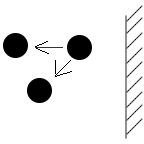# The Equation of State for Real Gases Part Three

## Intermolecular Forces

We can use equation 4 in the preceding article to help us derive the final form of the van der Waals equation of state for real gases. To recall, we found that

4) P(V/n – b) = RT.

We begin by considering intermolecular forces. Intermolecular forces have a behavior that is nonexistent at long distances, attractive at intermediate distances, and repulsive at short distances. Here, we take into account only their attractive forces, which are electrical in nature.

Molecules that are close can attract each other over certain ranges, and if the attraction is great enough gases condense into liquids. We saw in our discussion on the kinetic theory of gases that the kinetic energy of collisions affects the pressure that the molecules exert on the container walls. Under certain conditions, short-range intermolecular forces also contribute to wall pressure. How so?Because of weak electrical attraction, molecules that are near the container wall at the edge of the gas have a net force that tends to pull them back, thus lessening the number of molecules impacting the wall and hence the gas pressure. This pressure is reduced in proportion to the density of the molecules, namely those that reside in the surface layer as well as in the layer directly underneath. The layer that is directly underneath the surface contributes by exerting an inward force on the edge molecules, as seen in figure 1. The weak attraction that exists between the molecules is akin to that of Newton’s law of gravitation, and the effect is that the net pressure is reduced by a factor proportional to 1/V2.

Taking all this into account, we can replace P by an effective pressure

5) P (effective) = P + a/(V/n)2,

where a is a constant dependent on the amount of attraction between the molecules; it takes into account the overlap of their electron clouds. Substituting this into equation 4 above gives us

6) (P + a/(V/n)2)(V/n – b) = RT.

If we let V/n = the specific volume V, then we have:

7) (P + a/V2)(V – b) = RT.

Equations 6 and 7 are known as the van der Waals equation of state. The constants a and b are chosen to fit the experimental data for the gas under consideration. When a and b are zero, the equation reduces to that of the ideal gas law.

In the next part, we will see how to use this equation of state to plot isotherms.

## This post is part of the series: The Equation of State for Real Gases

A basic introduction to van der Waal’s equation of state for real gases.